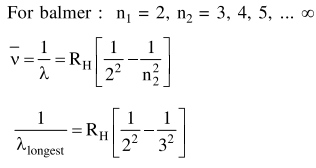Deepak Scored 45->99%ile with Bounce Back Crack Course. You can do it too!

# For the Balmer series in the spectrum of

Question:

For the Balmer series in the spectrum of $\mathrm{H}$

atom, $\quad \bar{v}=\mathrm{R}_{\mathrm{H}}\left\{\frac{1}{\mathrm{n}_{1}^{2}}-\frac{1}{\mathrm{n}_{2}^{2}}\right\}, \quad$ the correct

statements among (I) and (IV) are :

(I) As wavelength decreases, the lines in the series converge

(II) The integer $n_{1}$ is equal to 2

(III) The lines of longest wavelength corresponds to $\mathrm{n}_{2}=3$

(IV) The ionization energy of hydrogen can be calculated from wave number of these lines

1. (II), (III), (IV)

2. (I), (II), (III)

3. (I), (III), (IV)

4. (I), (II), (IV)

Correct Option: , 2

Solution: